# Pre Treatment & Physical Treatment: Aeration (Part - 2) Computer Science Engineering (CSE) Notes | EduRev

## Computer Science Engineering (CSE) : Pre Treatment & Physical Treatment: Aeration (Part - 2) Computer Science Engineering (CSE) Notes | EduRev

The document Pre Treatment & Physical Treatment: Aeration (Part - 2) Computer Science Engineering (CSE) Notes | EduRev is a part of the Computer Science Engineering (CSE) Course Environmental Engineering - Notes, Videos, MCQs & PPTs.
All you need of Computer Science Engineering (CSE) at this link: Computer Science Engineering (CSE)

REMOVAL OF VOCs BY AERATION IN COMPLETE STIRRED TANK REACTOR (CSTR) 

[A] VOC removal by surface aeration in CSTR

A mass balance for VOC in CSTR having surface aeration is given as: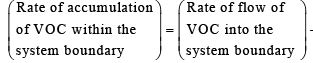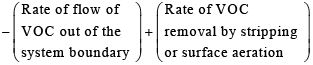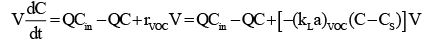(3.4.1)

Where, V is the volume of the CSTR (m3), dC/dt is the rate of change of VOC in the CSTR, Q is the liquid flow rate in and out of the reactor (m3/s), Cin is the VOC concentration in influent to the CSTR (µg/m3) and C is the VOC concentration in effluent from the CSTR (µg/m3). RVOC is the rate of VOC mass transfer (µg/m3s) and is given as: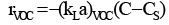(3.4.2)

Where, CS is the saturation concentration of VOC in the liquid (µg/m3) and (kLa)VOC is overall mass transfer coefficient (s-1) and it is determined using the oxygen mass transfer coefficient, (kLa)O2 using the following equation: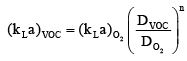(3.4.3)
Where, DVOC and DO2 are the diffusion coefficients of VOC and O2 in water, respectively (cm2/s) and n is an empirical constant.  Assuming steady state condition, V/Q=τ and CS=0, equation 1 becomes:(3.4.4)

Fraction of VOC removed=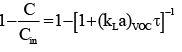(3.4.5)

[B] VOC removal by diffused aeration in CSTR

At steady state, mass balance on VOC in diffused aeration system in CSTR is given as: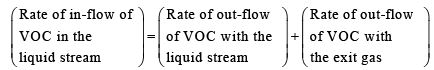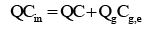(3.4.6)
Where, Qg is the diffused gas flow rate inside the CSTR (m3/s), Cg,e is the VOC concentration in exit gas (µg/m3). Bielefeldt and Stensel  gave the following relationship for Cg,e with C: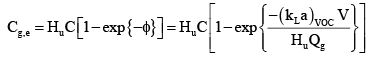(3.4.7)
Where, Hu=(H/RT) is the dimensionless value of Henry’s constant and f is VOC saturation parameter. After putting the value of Cg,e and rearranging, we get

Fraction of V OC removed =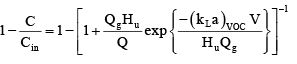(3.4.8)

Problem 3.4.1: Wastewater flow rate in a complete-mix activated sludge reactor having volume=2000 m3 and depth=8 m is 6000 m3/d. If the influent concentration of benzene is 200 µg/m3 and that the air flow rate (at standard condition) is 100 m3/min, determine the fraction of benzene that can be stripped off if the complete mix activated sludge reactor is equipped with

(a) surface aeration system
(b) diffused-air aeration system

Also given that: Oxygen diffusivity=2.11 × 10-5 cm2/s, benzene diffusivity=0.96 × 10-5 cm2/s, temperature=20 oC, n=1, Henry’s constant=5.49×10-3 m3atm/mol.

Solution: First the value of (kLa)VOC is determined.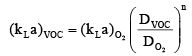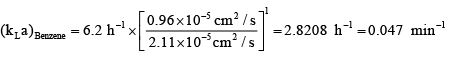Case a: Surface aeration system

t= ( V Q) = ( 2000 6000) = 0.333 d = 7.992 h

Fraction of VOC removed=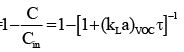Fraction of VOC removed =  1- 1 + 2.8208 x 7.992]-1 =0.9575

Case b: Diffused aeration system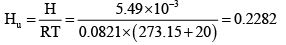We need gas flow rate at the actual condition i.e. at half of the tank depth (=4 m) and at 20 oC.  We know: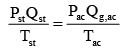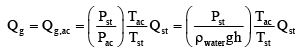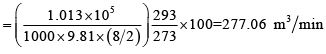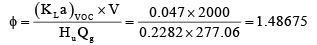Q=6000 m3/d = 4.1667 m3/min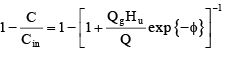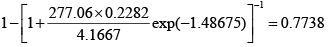PACKED TOWER AERATION

In packed tower aeration (PTA), wastewater to be treated in sprayed on the top of a tower. The tower is about 3-10 m in height and is packed with various types of packing which provide high surface area to volume ratio. Air is pumped simultaneously counter-currently through the packing from the bottom and removes the VOC from wastewater which itself in trickling over the packing. Air along with the VOC gets removed from the top while treated water is collected at the bottom.

Design of Packed-tower aeration unit

The height of the tower can be calculated using the following equation:

Z = HTU × NTU           (3.4.8)
Where, HTU is the height of transfer unit and NTU is the number of transfer units. HTU represents the rate of mass transfer for a particular type of packing. It determines the efficiency of mass transfer from liquid to gas phase. It is related to liquid loading rate and is given by: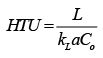(3.4.9)
Where, L is the ratio of superficial molar to mass liquid flow, KLa is the overall mass transfer coefficient; Co is the molar density of VOC in water (kmol/m3)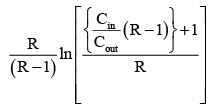(3.4.10)
Where, R=HuG/L is called the stripping factor, Hu is Henrys’ constant dimensionless, G=(QG/A) is the superfacial gas flow rate (kmol/h m2), A is the crosssectional area of packed bed (m2) and QG is the gas flow rate (kmol/h).

Offer running on EduRev: Apply code STAYHOME200 to get INR 200 off on our premium plan EduRev Infinity!

56 docs|57 tests

,

,

,

,

,

,

,

,

,

,

,

,

,

,

,

,

,

,

,

,

,

;